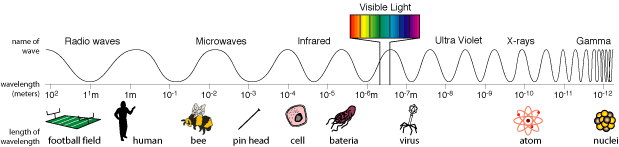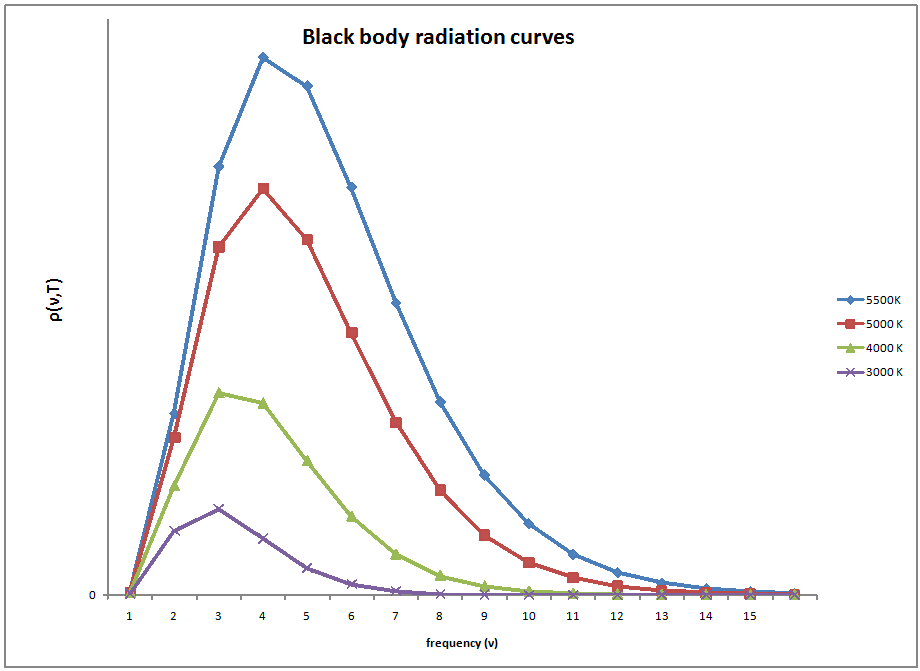$$\newcommand{\vecs}{\overset { \rightharpoonup} {\mathbf{#1}} }$$ $$\newcommand{\vecd}{\overset{-\!-\!\rightharpoonup}{\vphantom{a}\smash {#1}}}$$$$\newcommand{\id}{\mathrm{id}}$$ $$\newcommand{\Span}{\mathrm{span}}$$ $$\newcommand{\kernel}{\mathrm{null}\,}$$ $$\newcommand{\range}{\mathrm{range}\,}$$ $$\newcommand{\RealPart}{\mathrm{Re}}$$ $$\newcommand{\ImaginaryPart}{\mathrm{Im}}$$ $$\newcommand{\Argument}{\mathrm{Arg}}$$ $$\newcommand{\norm}{\| #1 \|}$$ $$\newcommand{\inner}{\langle #1, #2 \rangle}$$ $$\newcommand{\Span}{\mathrm{span}}$$ $$\newcommand{\id}{\mathrm{id}}$$ $$\newcommand{\Span}{\mathrm{span}}$$ $$\newcommand{\kernel}{\mathrm{null}\,}$$ $$\newcommand{\range}{\mathrm{range}\,}$$ $$\newcommand{\RealPart}{\mathrm{Re}}$$ $$\newcommand{\ImaginaryPart}{\mathrm{Im}}$$ $$\newcommand{\Argument}{\mathrm{Arg}}$$ $$\newcommand{\norm}{\| #1 \|}$$ $$\newcommand{\inner}{\langle #1, #2 \rangle}$$ $$\newcommand{\Span}{\mathrm{span}}$$$$\newcommand{\AA}{\unicode[.8,0]{x212B}}$$

Blackbody radiation is a cornerstone in the study of quantum mechanics.This experiment is what led to the discovery of a field that would revolutionize physics and chemistry. Quantum mechanics gives a more complete understanding of the fundamental mechanisms at the sub-atomic level.

## Introduction

The work done at the turn of the 20th century on blackbody radiation was the beginning of a totally new field of science. Blackbody radiation is a theoretical concept in quantum mechanics in which a material or substance completely absorbs all frequencies of light. Because of the laws of thermodynamics, this ideal body must also re-emit as much light as it absorbs.Although there is no material that can truly be a blackbody, some have come close.Carbon in its graphite form is about 96% efficient in its absorption of light.

The concept of blackbody radiation is seen in many different places.The intensity of the energy coming from the radiator is a function only of temperature. A good example of this temperature dependence is a flame. The flame starts out with a low frequency emitting red light in the visible range, as the temperature increases the flame turns white and then blue as is moves across the visible spectrum with an increasing temperature. Also, with each temperature corresponds a new maximum radiance which can be emitted. As the temperature increases, the total radiation emitted also increases due to an increase in the area under the curve.Electromagnetic spectrum:click for citation

Lord Rayleigh and J. H. Jeans developed an equation which explained blackbody radiation at low frequencies.The equation which seemed to express blackbody radiation was built upon all the known assumptions of physics at the time. The big assumption which Rayleigh and Jean implied was that infinitesimal amounts of energy were continuously added to the system when the frequency was increased. Classical physics assumed that energy emitted by atomic oscillations could have any continuous value. This was true for anything that had been studied up until that point, including things like acceleration, position, or energy.They came up with Rayleigh-Jeans law and the equation they derived was

$d\rho \left( \nu ,T \right) = \rho_{\nu} \left( T \right) d \nu = \frac{8 \pi k_B T}{c^3} \nu^2 d\nu$

Experimental data performed on the black box showed slightly different results than what was expected by the Rayleigh-Jeans law. The law had been studied and widely accepted by many physicists of the day, but the experimental results did not lie, something was different between what was theorized and what actually happens.The experimental results showed a bell type of curve but according to the Rayleigh-Jeans law the frequency diverged as it neared the ultraviolet region.This inconsistency was termed the ultraviolet catastrophe.

## Ultraviolet Catastrophe

During the 19th century much attention was given to the study of heat properties of various objects. An idealised model that was considered was the Black Body, an object which absorbs all incident radiation and then re-emits all this energy again. We can think of the radiating energy as standing waves inside our blackbody cavity. The energy of the radiating waves at a given frequency ν, should be proportional to the number of modes at this frequency. Classical physics states that all these modes have the same energy kT (a result derived from classical thermodynamics) and as the number of modes is proportional to $$\nu^2$$:

$E \propto \nu^2 kT \label{1.1.1}$

This implies that we would expect most of the energy at higher frequency, and this energy diverges with frequency. If we try and sum the energies at each frequency we find that there is an infinite energy in ths system! This paradox was called the ULTRAVIOLET CATASTROPHE.

It was left to Planck to resolve this gaping paradox, but postulated that the energy of the modes could only come in discrete packets – quanta – of energy:

$E = h\nu, 2h\nu, 3h\nu, \ldots \qquad \Delta E = h\nu \label{1.1.2}$

Using statistical mechanics Planck found that the modes at higher frequency were less likely excited so the average energy of these modes would decrease with the frequency. The exact expression for the average energy of each mode is given by the Planck distribution:

$\langle E \rangle = \frac{h\nu}{\exp(\frac{h\nu}{KT}) - 1} \label{1.1.3}$

You can see that if the frequency is low then the average energy tends towards the classical result, and as frequency goes to infinity we get that the average energy goes to zero as expected.

Max Planck was the first person to properly explain this experimental data. Rayleigh and Jean made the assumption that energy is continuous, but Planck took a slightly different approach. He said energy must come in certain unit intervals instead of being any random unit or number. He instead “quantized” energy in the form of $$E= nh\nu$$ where $$n$$ is an integer, $$h$$ is a constant, and $$\nu$$ is the frequency. This assumption proved to be the missing piece of the puzzle and Planck derived an expression which could explain the experimental data.

$d\rho \left( \nu ,T \right) = \rho_{\nu} \left( T \right) d\nu = \dfrac{8 \pi k_B T}{c^3} \dfrac{nu^2}{e^{hv/K_bT}-1} d\nu$

This now famous equation is known as the Planck Distribution Law for Blackbody Radiation.The h in this equation is the famous Planck’s constant, which has a value of $$6.626 \times 10^-34\; J \;s$$.Blackbody Radiation is shared under a CC BY-NC-SA 4.0 license and was authored, remixed, and/or curated by LibreTexts.